Payal Tandon
Co-founder, e-GMAT
Welcome to e-GMAT Support!
I am Payal, Co-Founder of e-GMAT.
Feel free to ask any Query.We will be contacting you soon on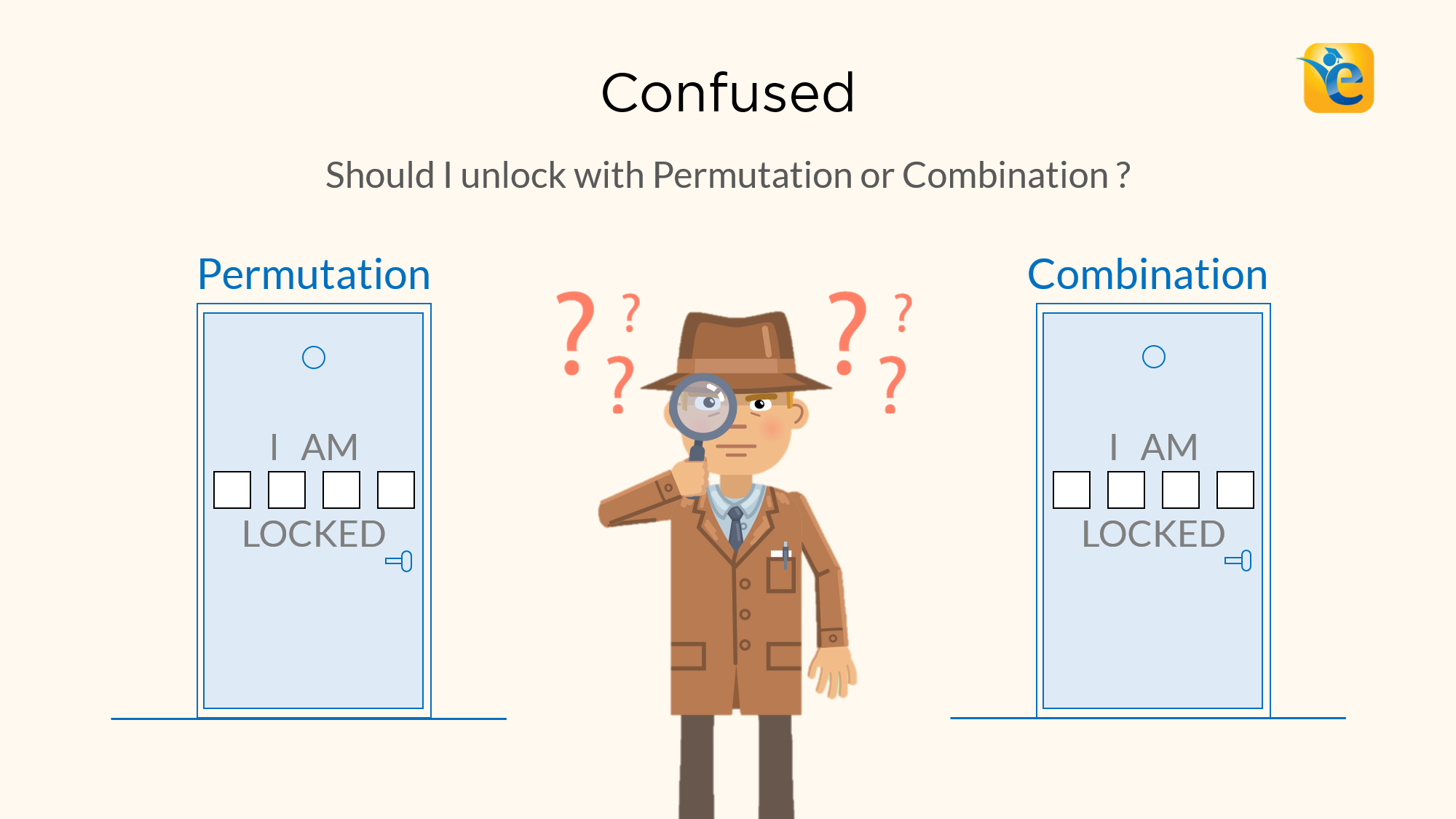# Difference between Permutation and Combination explained with examples

Permutations and Combinations is a topic full of conundrums. The biggest one is, understanding the difference between permutation and combination. Should I solve this...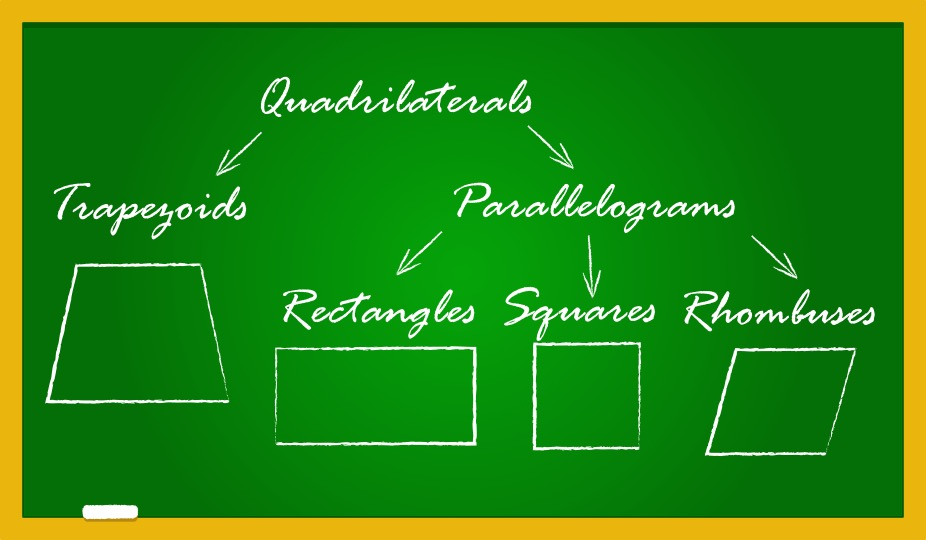# Properties of Quadrilaterals – Rectangle, Square, Parallelogram, Rhombus, Trapezium | 2023

In Euclidean geometry, a quadrilateral is a four-sided 2D figure whose sum of internal angles is 360°. The word quadrilateral is derived from two Latin words ‘quadri’ and...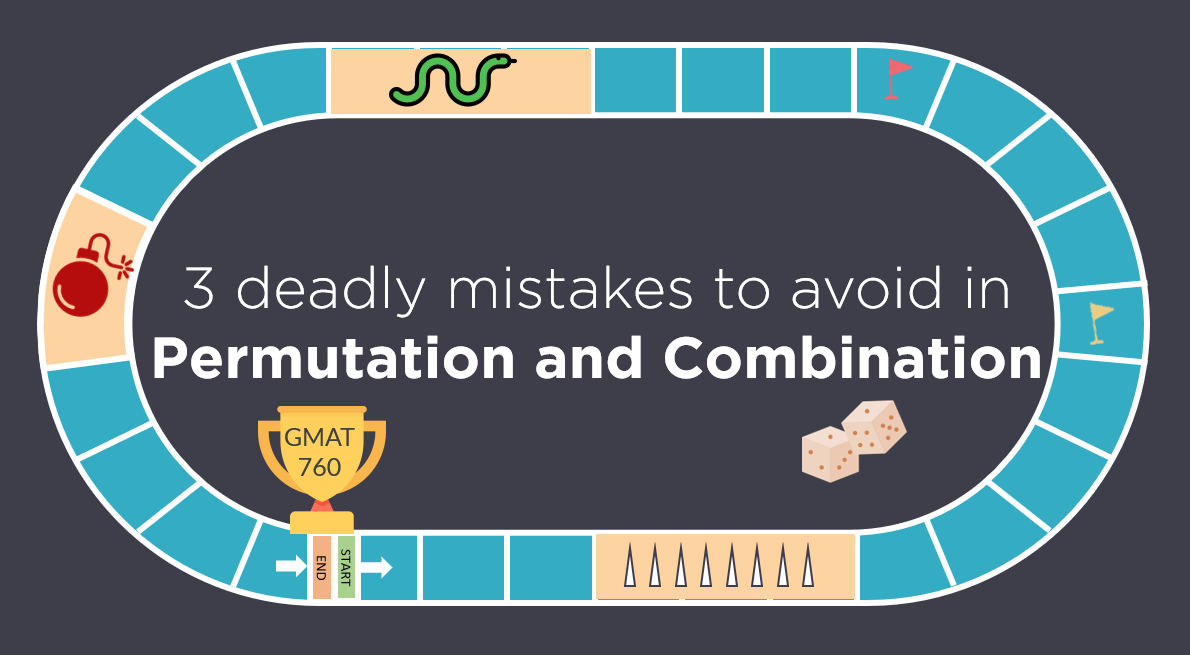# Permutation and Combination | Avoid these 3 Mistakes | GMAT Quant

Like any GMAT Quant topic, Permutation and Combination has its own traps. Most students fall in these traps and ultimately, are not able to secure their target GMAT score....# Difference between Permutation and Combination explained with examples

Permutations and Combinations is a topic full of conundrums. The biggest one is, understanding the difference between permutation and combination....# Properties of Quadrilaterals – Rectangle, Square, Parallelogram, Rhombus, Trapezium | 2023

In Euclidean geometry, a quadrilateral is a four-sided 2D figure whose sum of internal angles is 360°. The word quadrilateral is derived from two...# Permutation and Combination | Avoid these 3 Mistakes | GMAT Quant

Like any GMAT Quant topic, Permutation and Combination has its own traps. Most students fall in these traps and ultimately, are not able to secure...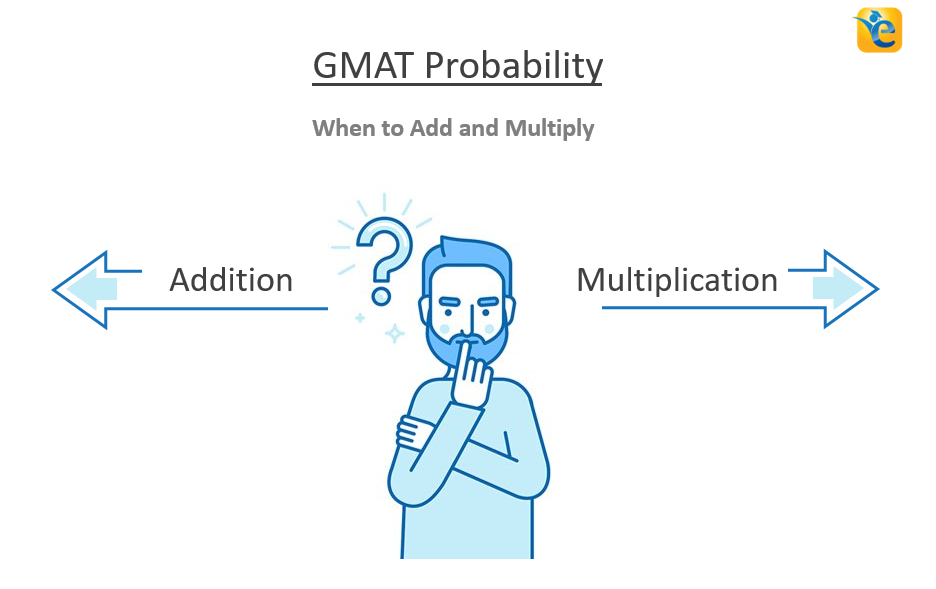# GMAT Permutation and Combination | When to Add and Multiply

Are you always confused whether to add or multiply the cases while solving GMAT permutation and combination questions? Do you often get questions...# Circle Formulas -What is a Circle and its properties? (Definition & Examples)

A circle is a closed shape formed by tracing a point that moves in a plane such that its distance from a given point is constant. The word circle is...

# More articles

## Difference between Permutation and Combination explained with examples

A 8 min read Permutations and Combinations is a topic full of conundrums. The biggest one is,...

## Properties of Quadrilaterals – Rectangle, Square, Parallelogram, Rhombus, Trapezium | 2023

A 9 min read In Euclidean geometry, a quadrilateral is a four-sided 2D figure whose sum of...

## Permutation and Combination | Avoid these 3 Mistakes | GMAT Quant

A 12 min read Like any GMAT Quant topic, Permutation and Combination has its own traps. Most...

## GMAT Permutation and Combination | When to Add and Multiply

A 10 min read Are you always confused whether to add or multiply the cases while solving GMAT...

## Circle Formulas -What is a Circle and its properties? (Definition & Examples)

A 8 min read A circle is a closed shape formed by tracing a point that moves in a plane such that...

## Lines and Angles – Definitions, Properties, Types, Practice Questions

A 8 min read Ancient mathematicians introduced the concept of lines to represent straight objects...

## If p, r, and s are consecutive integers in ascending order and x is the average (arithmetic mean) of the three integers – OG 2020 Question #304 with Solution

A 2 min read OG 2020: Question No. 304 If p, r, and s are consecutive integers in ascending order and x is the average (arithmetic mean) of the three integers, what is the value of x? Twice x is equal to the sum of p, r, and...

## Improve accuracy in GMAT Math questions on Polygons

A 10 min read A polygon is a two-dimensional closed figure made up of at least 3 line segments....

## GMAT Problem Solving – 3 mistakes you must avoid in Time and Work questions

A 10 min read GMAT problem solving questions on Time and Work can be solved easily and quickly provided you do not make mistakes while solving them. In the last article, we compared the conventional methods of solving Time and...

## GMAT Word Problems | Application of Average Speed in Time – Distance Problems

A 12 min read GMAT Word problems on Time, and Speed, and Distance are based on the simple formula of  D = S x T, where D is the distance, S is speed and T is time. These GMAT word problems might look easy at first but if...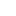###### Browse Related Topics# Achieve 740+on the GMAT in 30 days!Sign up for our free trial and get:400+ Practice questions with detailed solutions10+ hours of AI-driven video lessonsAdaptive mock test with ESR+ analysis